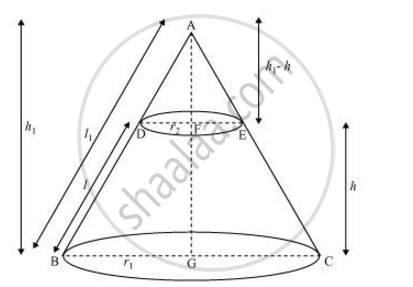Share

# Derive the Formula for the Curved Surface Area and Total Surface Area of the Frustum of Cone. - CBSE Class 10 - Mathematics

#### Question

Derive the formula for the curved surface area and total surface area of the frustum of cone.

#### SolutionLet ABC be a cone. A frustum DECB is cut by a plane parallel to its base. Let r1 and r2 be the radii of the ends of the frustum of the cone and h be the height of the frustum of the cone.

r_2/r_1 = (h_1-h)/h_1 =(l_1-l)/l_1

r_2/r_1 = 1- h/h_1 = 1 - 1/l_1

l - l/l_1= r_2/r_1

l/l_1 =1-r_2/r_1 =(r_1-r_2)/r_1

l_1/l = r_1/(r_1-r_2)

l_1 = (r_1l)/(r_1-r_2)

CSA of frustum DECB = CSA of cone ABC − CSA cone ADE

= pir_1l_1 - pir_2(l_1-l)

=pir_1((lr_1)/(r_1-r_2))-pir_2[(r_1l)/(r_1-r_2)-l]

= (pir_1^2l)/(r_1-r_2) - pir_2((r_1l-r_1l+r_2l)/(r_1-r_2))

=(pir_1^2l)/(r_1-r_2)-(pir_2^2l)/(r_1-r_2)

= pil[(r_1^2-r_2^2)/(r_1-r_2)]

CSA of frustum = Π(r1 + r2)l

Total surface area of frustum = CSA of frustum + Area of upper circular end + Area of lower circular end

= pi(r_1+r_2)l+pir_2^2+pir_1^2

=pi[(r_1+r_2)l+r_1^2+r_2^2]

Is there an error in this question or solution?

#### APPEARS IN

NCERT Solution for Mathematics Textbook for Class 10 (2019 to Current)
Chapter 13: Surface Areas and Volumes
Ex. 13.40 | Q: 6 | Page no. 258

#### Video TutorialsVIEW ALL 

Solution Derive the Formula for the Curved Surface Area and Total Surface Area of the Frustum of Cone. Concept: Frustum of a Cone.
S